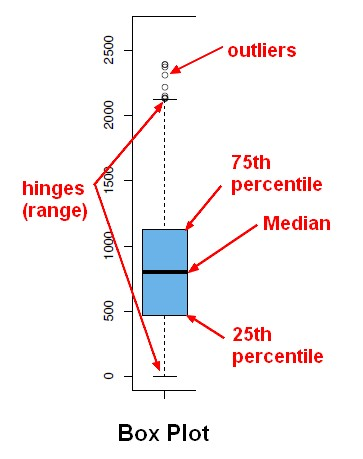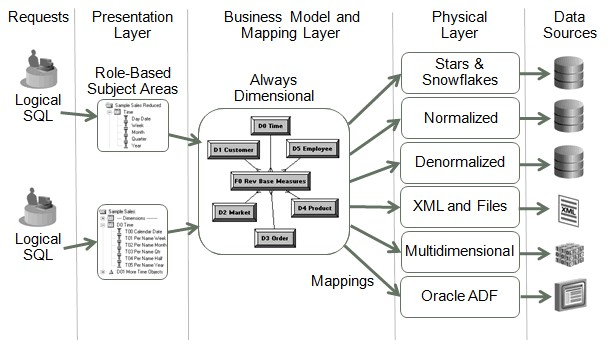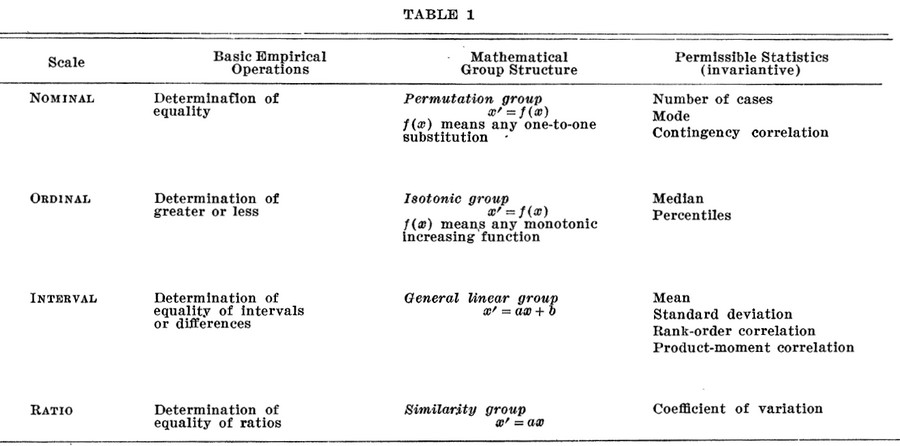# Quantile - (Median|Middle)

The median is a measure of center. The middle number of a set of data is the median.

This measure is resistant.

The median is a 50th percentile (or “middle” quartile). Half of the data is below the median.

## How to calculate a median ?

### With an uneven number of numbers

• Score: 7, 5, 8, 9, 5
• Ordered Score (after a sort): 5, 5, 7, 8, 9

The middle number is the third score, or 7, so the median of this data is 7.

### With an even number of numbers

• Score: 5, 8, 9, 5
• Ordered Score: 5, 5, 8, 9

When there is an even number of numbers, there is no true value in the middle.

The median is then the mean of the two middle numbers of (5 + 8)=13/2 = 6,5.

If there are four students sitting in a row, the middle of the row is halfway between the second and third students.

## Example

### Python

``````def median(sequence):
isEven = True
if len(sequence) % 2 == 0:
isEven = True
else:
isEven = False

sequence.sort()
# - 1
index_average = int(round(len(sequence)/2))

if isEven:
median = (sequence[index_average-1]+sequence[index_average])/2.00
else:
median = sequence[index_average]
return median```
```

## Documentation / Reference

Recommended PagesData Visualization - Box Plot

A box plot is a good summary of a distribution and was invented by John Tukey. See Five-number summary The boxplot is a special case of the quantile function in that it only returns the 1st, 2nd and...Distribution - Measures of (center|central tendency) (Mean, Median, Mode)

A Measure of central tendency is a measure that describes the middle or center point of a distribution. A good measure of central tendency is representative of the distribution. The mean, the median and...Distribution - Quantile Analysis

A quantile is a statistic that identifies the data that is less than the given value (ie that fall at or below a score in a distribution). A quantile function will always rank the data before giving any...Function - (Aggregate | Aggregation)

Aggregate functions return a single value calculated or selected from values that are in a aggregation relationship (ie a set) This values are also known as summary because they try to summarize...OBIEE - (Display Function|Derived Measures) (Rank, TopN, Median, )

Oracle BI Answers simplifies the use of derived measures i.e. measures that are computed on a query result set such as ranks, Ntiles, standard deviations, running totals, moving averages, and moving medians....Quantile - Functions

This page regroups all known quantile function. Specific Oracle function with Quantile. Oracle has also the ntile function. MEDIAN PERCENTILE_DISC (Oracle) is an inverse distribution function...Quantile - Quartile

A quartile is a specialized quantile. It divides the data into 4 approximately equal groups. The first and third quartile (or the 25th and 75th percentiles) are two very commonly used percentiles....Statistics - (Data|Data Set) (Summary|Description) - Descriptive Statistics

Summary are a single value summarizing a array of data. They are: selected or calculated through reduction operations. They are an important element of descriptive analysis One of the most important...Statistics - (Scales of measurement|Type of variables)

According to Stevens theory (On the Theory of Scales of Measurement), the four scales of measurement are: nominal ordinal ...